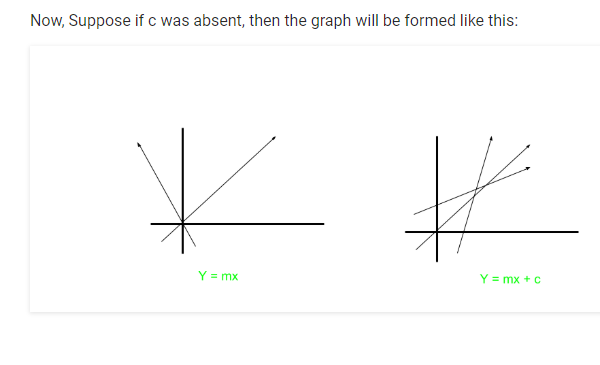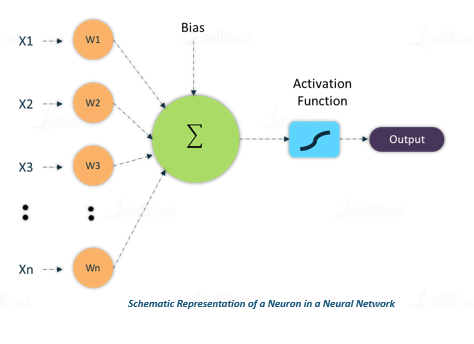2 views

I was having some issues regarding my backpropagating neural network for the XOR operator. I did a little more work and realized that it may have to do with not having a bias neuron.

My question is, what is the role of the bias neuron in general, and what is its role in a backpropagating neural network that recognizes the XOR operator? Is it possible to create one without a bias neuron?

by (108k points)

Bias is similar to the intercept added in a linear equation. It is an additional parameter in the Neural Network which is used to adjust the output along with the weighted sum of the inputs to the neuron. Thus, Bias is a constant which helps the model in a way that it can fit best for the given data.

The processing done by the neuron is:

output  = sum (weights * inputs) + bias

For example, consider an equation y=mx+c

Here m is acting as weight and the constant c is acting as bias.Due to the absence of bias, the model will train over point passing through origin only, which is not in accordance with a real-world scenario. Also with the foundation of bias, the model will become more flexible.

It helps the model to learn the patterns and it acts like an input node that always produces constant value 1 or other constant. Because of this property, they are not attached to the previous layer.

Bias units are not connected to any previous layer, it is just appended to the start/end of the input and each hidden layer, and is not affected by the values in the previous layer.

You can refer to this diagram:Here x1,x2,x3 are inputs and w1, w2,w3 are weights. It takes an input, processes it, passes it through an activation function, and returns the output.

The role of the bias neuron in the neural net that attempts to solve the XOR is to minimize the size of the neural net. Normally, for fundamental logic functions such as AND, OR, NAND, etc, you are trying to create a neural network with 2 input neurons, 2 hidden neurons, and 1 output neuron. This can't be done for XOR because the simplest way you can model an XOR is with two NANDs.

You can consider x and y as your input neurons, the gate in the middle is your "bias" neuron, the two gates following are your "hidden" neurons and finally you have the output neuron. You cannot solve XOR without having a bias neuron, as it would require that you increase the number of hidden neurons to a minimum of 3 hidden neurons. In that case, the 3rd neuron basically acts as a bias neuron.